• 2022-03-20 21:41:05

• 顾名思义，似然似然，即是可能Maybe好像，就是像某个东西的可能性。在统计学上定义为给定输出x时，关于参数θ的似然函数L(θ|x)（在数值上）等于给定参数θ后变量X的概率：L(θ|x)=P(X=x|θ)。

• 似然函数在推断统计学（Statistical inference）中扮演重要角色，如在最大似然估计和费雪信息之中的应用等等。“似然性”与“或然性”或“概率”意思相近，都是指某种事件发生的可能性，但是在统计学中，“似然性”和“或然性”或“概率”又有明确的区分。概率用于在已知一些参数的情况下，预测接下来的观测所得到的结果，而似然性则是用于在已知某些观测所得到的结果时，对有关事物的性质的参数进行估计。

  你们看懂了吗，反正我没弄清概率和似然的区别。所以我就都理解成概率


# 逻辑回归中的似然损失函数

• 逻辑回归为什么使用似然损失，而不是使用均方损失，大概是均方损失函数导出来梯度下降公式的十分丑陋，导致运算量巨大。

m i n E ( x ) = 1 n ∑ i = 0 n ( g ( w T x i ) − y i ) 2 minE(x)=\frac{1}{n}\sum_{i=0}^n(g(w^Tx_i)-y_i)^2
而逻辑回归函数又长这样 g ( w T x i ) = 1 1 + e w T x g(w^Tx_i)=\frac{1}{1+e^{w^Tx}}

外导一个2还可以，内导就恶心了，直接一个分式指数，不知道你们算这个心情如何，反正我是吃不下饭了。
∂ E ∂ w = − 1 n ∑ i = 0 n 2 ( 1 1 + e w T x − y i ) ( 1 + e w T x ) − 2 e w T x x i \frac{\partial E}{\partial w}=-\frac{1}{n}\sum_{i=0}^n2(\frac{1}{1+e^{w^Tx}}-y_i)(1+e^{w^Tx})^{-2}e^{w^Tx}x_i

这能忍吗，就算咱们忍的下去，cpu也忍不了啊，所以方差损失就被无情抛弃

然后需要一个更加美丽的损失函数登场了——似然函数

## 似然函数

• 首先得先知道逻辑回归是为了解决01问题
知道这个我们就能三下五除二写出它似然函数的一小小块

P ( y i ∣ x i ; w ) = y i P ( y i = 1 ∣ x i ; w ) + ( 1 − y i ) P ( y i = 0 ∣ x i ; w ) P(y_i|x_i;w)=y_iP(y_i=1|x_i;w)+(1-y_i)P(y_i=0|x_i;w)

1. y i = 1 y_i=1 就是左边那个 P ( y i = 1 ∣ x i ; w ) P(y_i=1|x_i;w) 有效，
2. y i = 0 y_i=0 就是右边那个 P ( y i = 0 ∣ x i ; w ) P(y_i=0|x_i;w) 有效

哇,小小一个 y i y_i 竟然有如此妙用，当然这一切都建立在 y i y_i 只能取0或1，要取个0到1就麻烦了.
然后问题来了，这个 P ( y i = 1 ∣ x i ; w ) P(y_i=1|x_i;w) 是啥子，从外表来看，它是条件概率，在x、w的取值基础上 y i = 1 y_i=1 的概率。而我们的对象是逻辑回归，逻辑回归函数又不能从其他地方引入，并且逻辑回归的值恰好为0到1，这很概率，所以从此引入逻辑回归函数就很舒服

P ( y i = 1 ∣ x i ; w ) = 1 1 + e w T x P(y_i=1|x_i;w)=\frac{1}{1+e^{w^Tx}}
P ( y i = 0 ∣ x i ; w ) = 1 − 1 1 + e w T x = e w T x 1 + e w T x P(y_i=0|x_i;w)=1-\frac{1}{1+e^{w^Tx}}=\frac{e^{w^{T}x}}{1+e^{w^Tx}}

那么现在就将所有案例的概率值都累乘起来就是最终似然函数的形态了。
L = ∏ i = 0 n P ( y i ∣ x i ; w ) L=\prod_{i=0}^{n}P(y_i|x_i;w)
不要忘记我们的目的——求出梯度下降的公式。
一级展开：
L = ∏ i = 0 n ( y i P ( y i = 1 ∣ x i ; w ) + ( 1 − y i ) P ( y i = 0 ∣ x i ; w ) ) L=\prod_{i=0}^{n}(y_iP(y_i=1|x_i;w)+(1-y_i)P(y_i=0|x_i;w))
二级展开：
L = ∏ i = 0 n ( y i 1 1 + e w T x i + ( 1 − y i ) e w T x 1 + e w T x ) L=\prod_{i=0}^{n}(y_i\frac{1}{1+e^{w^Tx_i}}+(1-y_i)\frac{e^{w^{T}x}}{1+e^{w^Tx}})
然后同分母合并一下
L = ∏ i = 0 n ( y i + ( 1 − y i ) e w T x i ) 1 1 + e w T x i L=\prod_{i=0}^{n}(y_i+(1-y_i)e^{w^Tx_i})\frac{1}{1+e^{w^Tx_i}}

还是有点复杂，我们再用对数化，把分子分母分开：
l n L = ∑ i = 0 n [ l n ( y i + ( 1 − y i ) e w T x i ) − l n ( 1 + e w T x i ) ] lnL=\sum_{i=0}^{n}[ln(y_i+(1-y_i)e^{w^Tx_i})-ln(1+e^{w^Tx_i})]

这个时候在观察一下
左边的东西，似乎有些有趣的性质，

l n ( y i + ( 1 − y i ) e w T x i ) = { l n y i = 0 , y i = 1 l n e w T x i = w T x i , y i = 0 ln(y_i+(1-y_i)e^{w^Tx_i})=\left\{ \begin{aligned} &lny_i=0, &&y_i=1 \\ & lne^{w^Tx_i}=w^Tx_i ,&& y_i=0 \end{aligned} \right. ,
那么，就可以将其简化一下。
l n ( y i + ( 1 − y i ) e w T x i ) = ( 1 − y i ) w T x i ln(y_i+(1-y_i)e^{w^Tx_i})=(1-y_i)w^Tx_i

简化完成

带入原来的式子
l n L = ∑ i = 0 n [ ( 1 − y i ) w T x i − l n ( 1 + e w T x i ) ] lnL=\sum_{i=0}^{n}[(1-y_i)w^Tx_i-ln(1+e^{w^Tx_i})]

然后呢就可以开始求w的偏导了
∂ ( l n L ) ∂ w = ∑ i = 0 n [ ( 1 − y i ) x i − x i 1 + e w T x i ] = ∑ i = 0 n [ ] \frac{\partial (lnL)}{\partial w}=\sum_{i=0}^{n}[(1-y_i)x_i-\frac{x_i}{1+e^{w^Tx_i}}] =\sum_{i=0}^{n}[]

剩下的交给你们…

更多相关内容
• 由于是损失，因此对数似然函数的取值越小，则证明概率估计越准确，模型越理想。需要注意的是，对数损失只能用于评估分类型模型。 为了计算对数损失，分类器必须提供对输入的所属的每个类别的概率值，不只是最可能的...

除了上节的布里尔分数外，另一种常用的概率损失衡量是对数损失（log_loss），又叫做对数似然、逻辑损失或者交叉熵损失，它是多元逻辑回归以及一些拓展算法（比如神经网络）中使用的损失函数。它被定义为，对于一个给定的概率分类器，在预测概率为条件的情况下，真实概率发生的可能性的负对数。由于是损失，因此对数似然函数的取值越小，则证明概率估计越准确，模型越理想。需要注意的是，对数损失只能用于评估分类型模型。

为了计算对数损失，分类器必须提供对输入的所属的每个类别的概率值，不只是最可能的类别。对数损失函数的计算公式如下：

其中，Y为输出变量，X为输入变量，N为样本数，M为可能的类别数，yij表示类别j是否是输入实例xi的真实类别，pij为模型或分类器预测输入实例xi属于类别j的概率。

如果只有两类{0，1},则对数损失函数可以简化为

还是以手写数据集为例，看一下贝叶斯、逻辑回归以及支持向量机的表现：

from sklearn.datasets import load_digits
from sklearn.model_selection import train_test_split
from sklearn.naive_bayes import GaussianNB
from sklearn.svm import SVC
from sklearn.linear_model import LogisticRegression as LR
from sklearn.metrics import log_loss

X = data.data
y = data.target

Xtrain, Xtest, Ytrain, Ytest = train_test_split(X, y, test_size=0.3, random_state=420)

#朴素贝叶斯分类器
estimators = [GaussianNB().fit(Xtrain, Ytrain)
, LR(solver='lbfgs', max_iter=3000, multi_class='auto').fit(Xtrain,Ytrain)
, SVC(kernel='rbf', probability=True).fit(Xtrain,Ytrain)
]
name = ['GaussianNB', 'LogisticRegression', 'SVC']
for i, estimator in enumerate(estimators):
if hasattr(estimator, 'predict_proba'):
prob = estimator.predict_proba(Xtest)
print("{}'s log_loss is: {}\n".format(name[i], log_loss(Ytest, prob)))

得到结果如下所示：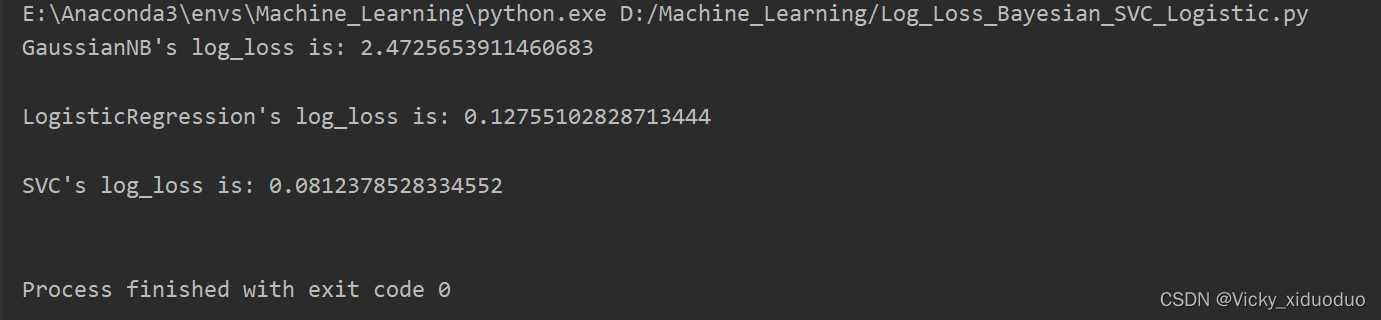对于概率类算法专用评估指标，贝叶斯的分数是最差的，按道理来说，贝叶斯模型是纯概率模型，在概率模型的评估指标上应该优于支持向量机这样的非概率模型。

这是因为逻辑回归和SVC都是以最优化为目的来求解模型，然后进行分类的算法。而朴素贝叶斯中，却没有最优化的过程。对数似然函数直接指向模型最优化的方向，甚至就是逻辑回归本身的损失函数，因此在逻辑回归和SVC上表现得更好。

那如何在更换贝叶斯模型的前提下，减少损失？答：调解概率的校准程度。校准程度越高，模型对概率的预测越准确，算法在做判断时就越有自信，模型就会更稳定。如果我们追求模型在概率预测上必须尽量贴近真实概率，那我们就可以使用可靠性曲线（Reliabiltiy Curve）来调节概率的校准程度。

可靠性（Reliabiltiy Curve）曲线将在下节中进行介绍。

展开全文算法 深度学习 人工智能
• 该文章对负对数似然函数进行了解释，有公式有代码，然后大彻大悟。这里的负对数似然损失就是交叉熵函数。 负对数似然公式：[损失函数]——负对数似然 - 簡書 (jianshu.com) 交叉熵公式：(4条消息) 交叉熵损失...

看论文，论文中写模型最后用的损失函数是交叉熵函数，但代码却是torch.nn.functional.nll_loss

很是不理解，但后来看到一篇博客：[损失函数]——负对数似然 - 簡書 (jianshu.com)

该文章对负对数似然函数进行了解释，有公式有代码，然后大彻大悟。这里的负对数似然损失就是交叉熵函数

负对数似然公式：[损失函数]——负对数似然 - 簡書 (jianshu.com)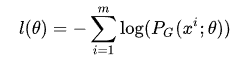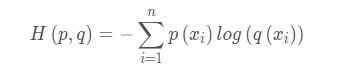而p(x)代表真实分布，取 0 或 1，取0时，与预测概率q(x)相乘还是0，取 1 时，可以省略，那么该式就等价于负对数似然函数。

pytorch中，对负对数似然损失函数有两种写法：

#1
torch.nn.NLLLoss(weight=None, size_average=None, ignore_index=-100, reduce=None, reduction='mean')
#Input: (N,C) , C是类别的个数
#Target: (N) ， target中每个值的大小满足 0 <= targets[i] <= C-1

#2
torch.nn.functional.nll_loss(input, target, weight=None, size_average=True)
#input - (N,C) C 是类别的个数
#target - (N) 其大小是 0 <= targets[i] <= C-1
#weight (Variable, optional) – 一个可手动指定每个类别的权重。

但需要注意的是，需要将输入通过Logsoftmax函数得到输出概率后再进行负对数似然损失函数计算。而pytorch中的交叉熵损失函数：

torch.nn.CrossEntropyLoss(weight=None, size_average=None, ignore_index=- 100, reduce=None, reduction='mean', label_smoothing=0.0)
torch.nn.functional.cross_entropy(input, target, weight=None, size_average=True)

Pytorch官方文件：Note that this case（torch.nn.CrossEntropyLoss） is equivalent to the combination of LogSoftmax and NLLLoss.

其他分类损失函数：

MSE: 均方误差，∑（预测值－真实值）²

效果还可以，但是通过sigmoid/softmx得到概率，再计算MSE损失函数，然后梯度下降更新参数，这样模型在训练初始阶段会学习得非常慢。损失函数｜交叉熵损失函数 - 知乎 (zhihu.com)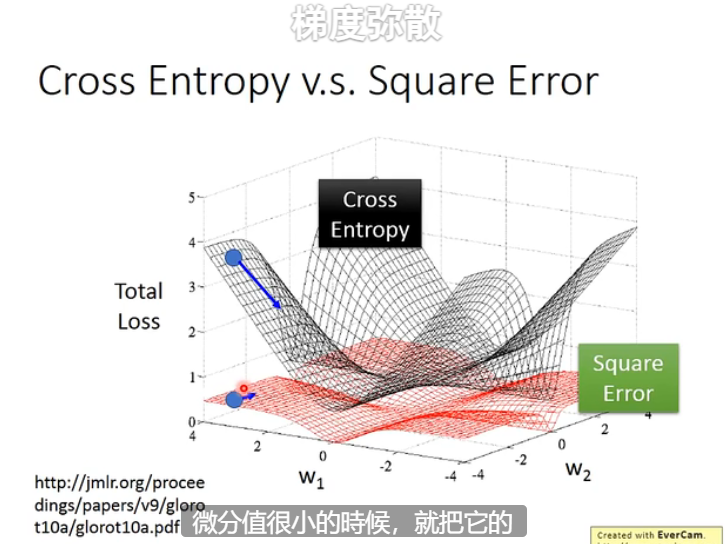李宏毅老师机器学习的这张课件，可以说明为什么平方差损失的训练会困难，因为梯度在非最优解的地方也很平坦，微分值很小，参数更新的步长会变小，难以到达最优。

展开全文python pytorch
• 对数似然对数似然 1. 似然 在开始之前需要区分一个知识：似然(likelihood)和概率(probability)。概率是一个事件发生的可能性，而似然指的是影响概率的未知参数。也就是说，概率是在该未知参数已知的情况下所得到...

阅读本文可以了解如下内容：

1. 似然
2. 似然估计
3. 对数似然
4. 负对数似然

#### 1. 似然

在开始之前需要区分一个知识：似然(likelihood)和概率(probability)。概率是一个事件发生的可能性，而似然指的是影响概率的未知参数。也就是说，概率是在该未知参数已知的情况下所得到的结果；而似然是该参数未知，我们需要根据观察结果，来估计概率模型的参数。

用数学方法可以描述为：
假设 X X 是离散随机变量，其概率质量函数 p p 依赖于参数$\theta$，则：
L ( θ ∣ x ) = p θ ( x ) = P θ ( X = x ) L(\theta|x)=p_{\theta}(x)=P_{\theta}(X=x)
其中 L ( θ ∣ x ) L(\theta|x) 为参数 θ \theta 的似然函数， x x 为随机变量 X X 的某一值。1

如果我们发现：
L ( θ 1 ∣ x ) = P θ 1 ( X = x ) > P θ 2 ( X = x ) = L ( θ 2 ∣ x ) L(\theta_{1}|x)=P_{\theta_{1}}(X=x)>P_{\theta_{2}}(X=x)=L(\theta_{2}|x)
那么似然函数就可以反映出这样一个朴素推测：在参数 θ 1 \theta_{1} 下随机变量 X X 取到 x x 的可能性大于在参数 θ 2 \theta_{2} 下随机向量 X X 取到 x x 的可能性。换句话说，我们更有理由相信，相对于 θ 2 \theta_2 θ 1 \theta_{1} 更有可能是该概率模型的真实参数。2

综上，概率(密度)表达给定 θ \theta 下样本随机向量 X = x X=x 可能性，而似然表达了给定样本 X = x X=x 下参数 θ 1 \theta_{1} (相对于另外的参数 θ 2 \theta_{2} )为真实值的可能性。我们总是对随机变量的取值谈概率，而在非贝叶斯统计的角度下，参数是一个实数而非随机变量，所以我们一般不谈一个参数的概率

#### 2. 最大似然估计(Maximum Likelihood Estimation, MLE)

假设我们有一个非常复杂的数据分布 P d a t a ( x ) P_{data}(x) ，但是我们不知道该分布的数学表达形式，所以我们需要定义一个分布模型 P G ( x ; θ ) P_{G}(x;\theta) ，该分布由参数 θ \theta 决定。
我们的目标是求得参数 θ \theta 使得定义的分布 P G ( x ; θ ) P_{G}(x;\theta) 尽可能的接近 P d a t a ( x ) P_{data}(x)

下面我们来看看最大似然估计如何操作：

1. P d a t a ( x ) P_{data}(x) 中采集m个样本 x 1 , x 2 , . . . , x m {x_{1},x_{2},...,x_{m}}
2. 计算样本的似然函数 L = ∏ i = 1 m P G ( x i ; θ ) L=\prod_{i=1}^{m}P_{G}(x^{i};\theta)
3. 求使得似然函数 L L 最大的参数 θ \theta : θ ∗ = arg ⁡ max ⁡ θ ∏ i = 1 m P G ( x i ; θ ) \theta^{*}=\arg \max _{\theta}\prod_{i=1}^{m} P_{G}(x^{i};\theta)

当来自 P d a t a ( x ) P_{data}(x) 的样本 x 1 , x 2 , . . . , x m {x_{1},x_{2},...,x_{m}} P G ( x ; θ ) P_{G}(x;\theta) 分布模型出现的概率越高，也就是 ∏ i = 1 m P G ( x i ; θ ) \prod_{i=1}^{m}P_{G}(x^{i};\theta) 越大， P G ( x ; θ ) P_{G}(x;\theta) P d a t a ( x ) P_{data}(x) 越接近。3

#### 3. 对数似然

在知道了最大似然估计之后就容易理解对数似然了。从公式可以看到，似然函数是很多个数相乘的形式： L = ∏ i = 1 m P G ( x i ; θ ) L=\prod_{i=1}^{m}P_{G}(x^{i};\theta)
然而很多数相乘并不容易计算，也不方便求导。如果我们对它取对数，连乘就会变成连加，计算起来要方便的多，求导也变得更加容易。
l ( θ ) = ∑ i = 1 m log ⁡ ( P G ( x i ; θ ) ) l(\theta)=\sum_{i=1}^{m}\log (P_{G}(x^{i};\theta))
这样一来就变成了我们非常熟悉的求极值过程， θ \theta 为自变量，我们需要找到某一个 θ \theta ，使得 l ( θ ) l(\theta) 最大。只需对 θ \theta 求导然后令导数等于0即可。
最大似然估计的一般步骤如下:
(1) 写出似然函数;
(2) 对似然函数取对数,得到对数似然函数;
(3) 求对数似然函数的关于参数组的偏导数,并令其为0,得到似然方程组;
(4) 解似然方程组,得到参数组的值。

#### 4. 负对数似然(Negative log-likelihood, NLL)1

由于对数似然是对概率分布求对数，概率 P ( x ) P(x) 的值为 [ 0 , 1 ] [0,1] 区间，取对数后为 ( − ∞ , 0 ] (-\infty ,0] 区间。再在前面加个符号，变成 [ 0 , ∞ ) [0,\infty) 区间。
l ( θ ) = − ∑ i = 1 m log ⁡ ( P G ( x i ; θ ) ) l(\theta)=-\sum_{i=1}^{m}\log (P_{G}(x^{i};\theta))
**写到这你有没有发现，这个公式不就是交叉熵吗？**只是少了一项 p ( x i ) p(x_{i}) ，但是真实标签的概率为1，所以省掉了。
关于交叉熵的理解可以参考我的另一篇博客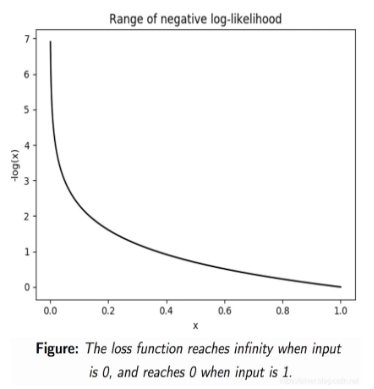我们期望似然估计越大越好，取完负号之后就是负对数似然越小越好，因此负对数似然函数可以作为损失函数。

Pytorch中对应的负对数似然损失函数为：

torch.nn.NLLLoss(weight=None, size_average=None, ignore_index=-100, reduce=None, reduction='mean')


值得注意的是，在使用该损失函数的时候并不需要将标签转换成one-hot形式，c类标签，就用c-1个数表示即可。
输入与输出数据的形状：

• input: (N,C)
• output:(N)
其中N为batch size，C为分类数量。假设batch size为3，有5个类别。我们用一段代码看看torch.nn.NLLLoss()如何使用：
>>> import torch
>>> m = torch.nn.LogSoftmax(dim=1)
>>> loss = torch.nn.NLLLoss()
>>> input
tensor([[ 0.1076, -1.4376, -0.6307,  0.6451, -1.5122],
[ 1.5105,  0.7662, -1.7587, -1.4581,  1.1357],
[-1.4673, -0.5111, -0.0779, -0.7404,  1.4447]], requires_grad=True)
>>> target = torch.tensor([1, 0, 4])
>>> output = loss(m(input), target)
>>> output.backward()


计算结果为：

tensor(1.3537)


实际上这段代码计算的是交叉熵，因为在pytorch交叉熵的官方文档中写道4

This criterion (CrossEntropyLoss) combines LogSoftmax and NLLLoss in one single class.

它先将输入经过log softmax函数，得到一个输出：

>>> data = nn.LogSoftmax(dim=1)(input)
>>> data
tensor([[-1.2812, -2.8264, -2.0195, -0.7437, -2.9010],
[-0.8118, -1.5561, -4.0810, -3.7804, -1.1866],
[-3.3349, -2.3787, -1.9455, -2.6080, -0.4229]])


然后根据负对数似然估计的公式，得到如下等式：
L o s s = − ( − 2.8264 − 0.8118 − 0.4229 ) / 3 = 1.3537 Loss = - (-2.8264 - 0.8118 - 0.4229 )/3 = 1.3537
和函数计算结果相同。

值得注意的是，nn.NLLLoss()函数虽然叫负对数似然损失函数，但是该函数内部并没有像公式里那样进行了对数计算，而是在激活函数上使用了nn.LogSoftmax()函数，所以nn.NLLLoss()函数只是做了求和取平均然后再取反的计算，在使用时要配合logsoftmax函数一起使用，或者直接使用交叉熵损失函数。

展开全文人工智能 算法
• ## 对数似然函数理解

万次阅读 2019-09-19 21:08:20
对数似然函数（log likelihood） 机器学习里面，对模型的训练都是对Loss function进行优化，在分类问题中，我们一般使用最大似然估计（Maximum likelihood estimation）来构造损失函数。对于输入的x，其对应的类...
• 深度学习以及机器学习中都会用到SoftMax函数，交叉熵损失函数与熵，对数似然函数等一些数学方面的知识，此文作为个人学习笔记。 1.softmax函数 （1）定义 多分类问题中，我们可以使用SoftMax函数，对输出的值归一...
• 参考资料： 极大似然估计 —— Maximum Likelihood Estimation Negative log likelihood explained 深度理解机器学习中常见损失函数 交叉熵、相对熵和负对数似然的理解深度学习 数学基础
• 4.4.2 **交叉熵代价函数（cross-entropy）**：4.4.3**对数似然代价函数（log-likelihood cost）**：5. 损失函数5.1 什么是损失函数5.2 常见的损失函数5.3 逻辑回归为什么使用对数损失函数5.4 对数损失函数是如何度量...
• 今天复习Logistic回归的时候涉及到二项分布的求最大似然解，突然发现这个对数似然函数熟悉，似曾相识，不就是交叉熵损失函数么，难道这仅仅是巧合，不能够。先看下这个推导。 1. 二项分布（0-1分布）： 2. 最大...
• 似然函数，在机器学习的算法模型中，可谓是屡见不鲜了，每次总觉得自己已经掌握了这个概念，但是遇到具体的情况后，发现还是很难说清楚，于是根据wiki上关于Likelihood function的解释，以及个人的学习理解，整理...极大似然估计
• 文章目录softmax神经元log-likelihood 损失函数 softmax神经元 softmax神经元的想法其实就是位神经网络定义一种新式的输出层，开始时和S型神经元一样，首先计算带权输入zjL=∑kwjkLakL−1+bjLz_j^L=\sum_kw_{jk}...深度学习 人工智能
• 本文主要介绍CTR场景中的对数损失函数和交叉熵损失函数，以及对数损失函数的取值范围，如果觉得对你有帮助，文末「点赞」来一个！极大似然估计在统计学领域，有两种对立的思想学派：贝叶斯学派和经典学派(频率学派)...
• 对数似然回归(Logistic)对泰坦尼克号幸存者分类 Logistic Regression 即为对数似然回归，它可以看做是一个最简单的人工神经网络。它是通过对数据进行拟合，从而选择一条线（超平面）将数据集分成两个部分，从而实现...python 深度学习 Logistic
• 形式一样，推导过程与代表意义不同 ... 在我重新抱起概率统计的课本之前，我一直都不清楚似然函数为什么是那样子的，...”“机器学习中的似然函数怎么看起来跟概率统计课本里的不一样呢？”“学长学长，我把这个m...
• 对数损失, 即 对数似然损失(Log-likelihood Loss) , 也称 逻辑斯谛回归损失(Logistic Loss) 或 交叉熵损失(cross-entropy Loss) , 是在概率估计上定义的.它常用于(multi-nominal, 多项)逻辑斯谛回归和神经网络,以及...
• 交叉熵 vs 二阶Loss函数 逻辑回归一些简单的网络中，我们会使用...ANN被设计的一个最大优势在于可以根据误差进行学习来调整参数。误差越大，则希望调整的幅度越大，从而收敛速度越快。而二阶loss函数则有可能误差越大计算机视觉 神经网络
• 文章目录1、最大似然估计的一般理论2、最大似然估计的性质3、最大似然估计推导逻辑回归的损失函数4、线性回归的损失函数5、小结 1、最大似然估计的一般理论 我们希望可以有些准则可以让我们从不同的模型中得到特定...逻辑回归 线性回归
• (1) 线性回归方程、(2) 损失函数推导、(3) 似然函数、(4) 三种梯度下降方法 1.概念简述 线性回归是通过一个或多个自变量与因变量之间进行建模的回归分析，其特点为一个或多个称为回归系数的模型参数的线性组合。如...python 回归
• 这让之前没怎么接触过似然函数的我，感到非常费解。本着简单的原则，从 本质思想 上梳理了下二者的关系，就我自己而言，理解起来方便多了。首先看 伯努利分布下，似然函数的意义： 在知道模型和样本的前提下，求出 ...
• 机器学习——损失函数前言一、分类问题的损失函数1、0-1损失（one-zero loss）2、Log Loss3、Focal Loss4、相对熵、KL散度（Relative Entropy/Kullback-Leibler Divergence）5、指数损失（Exponential Loss）6、...
• 机器学习常见损失函数 损失函数作用：损失函数是用于衡量模型预测值与真实值之间差距的函数损失函数的值越小越好。 常见的损失函数有如下几种： 分类问题 0-1损失函数（Zero-one Loss）： 当且仅当预测为真的时候...深度学习 人工智能
• ## 负对数似然(negative log-likelihood)

万次阅读 多人点赞 2019-03-30 16:32:28
文章目录negative log likelihood似然函数(likelihood function)OverviewDefinition离散型概率分布(Discrete probability distributions)连续型概率分布(Continuous probability distributions)最大似然估计(Maximum...最大似然估计
• 本文为机器学习基础 第一篇2020这个充满变化的不平凡的一年过去了，孕育着希望和机遇的2021即将到来，在此祝愿所有朋友幸福美满，蒸蒸日上，心想事成，欢喜如意，新年快乐！最近比较忙，好长...人工智能 深度学习 数据挖掘 编程语言
• 解决一个机器学习问题主要有两部分：数据和算法。而算法又有三个部分组成：假设函数损失函数、算法优化。我们一般在看算法书或者视频教学时，更多的是去推算或者说参数估计出其假设函数，而往往不太注重损失函数，...
• 统计学中，似然函数是一种关于统计模型参数的函数。给定输出x时，关于参数θ的似然函数L(θ|x)（在数值上）等于给定参数θ后变量X的概率：L(θ|x)=P(X=x|θ)。 似然函数在推断统计学（Statistical inference）中...
• 机器学习（第三章）3.3对数几率回归 1、对数几率回归的机器学习三要素 1.模型：根据具体问题，确定假设空间——此篇为线性模型，输出值范围为[0,1]，为近似阶跃的单调可微函数； 2.策略：根据评价标准，确定选取最优...回归
• 熵、交叉熵及似然函数的关系1. 熵1.1 信息量信息量：最初的定义是信号取值数量m的对数为信息量$$I$$,即 $$I=log_2m$$。这是与比特数相关的，比如一个信号只有两个取值，那么用1个bit便能将其表示。后来著名的香农...
• 我们知道，在机器学习中的监督学习算法里，在假设空间中构造一个决策函数 f，对于给定的输入 X，相应的输出 image 与原先的预期值 Y 可能不一致。于是，我们需要定义一个损失函数（Loss Function）来度量这...python 人工智能 深度学习 开发语言
• 1）面试官可能会问你平时常见的损失函数，并问你为啥要用这种函数来做训练呢？你看似常用的，但是面试官就是要问你为啥这么干 2）那就需要把各种损失函数的目的搞清楚，咱们用它干嘛，优化模型的参数更新，一个是...深度学习 人工智能...